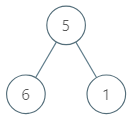# LeetCode: 1120. 子树的最大平均值¶

## 1、题目描述¶输入：[5,6,1]



• 树中的节点数介于 15000之间。
• 每个节点的值介于 0100000 之间。
• 如果结果与标准答案的误差不超过 $10^{-5}$，那么该结果将被视为正确答案。

## 2、解题思路¶

• DFS
• 自底向上反馈节点数量与和值，在当前节点计算平均值并更新结果
# Definition for a binary tree node.
# class TreeNode:
#     def __init__(self, x):
#         self.val = x
#         self.left = None
#         self.right = None

class Solution:
def maximumAverageSubtree(self, root: TreeNode) -> float:
ans = float('-inf')

def dfs(node):
nonlocal ans
if not node:
return 0, 0
left_sum, left_count = dfs(node.left)
right_sum, right_count = dfs(node.right)
cur_sum = left_sum + right_sum + node.val
cur_count = left_count + right_count + 1
ans = max(ans, cur_sum / cur_count)
return cur_sum, cur_count

dfs(root)
return ans